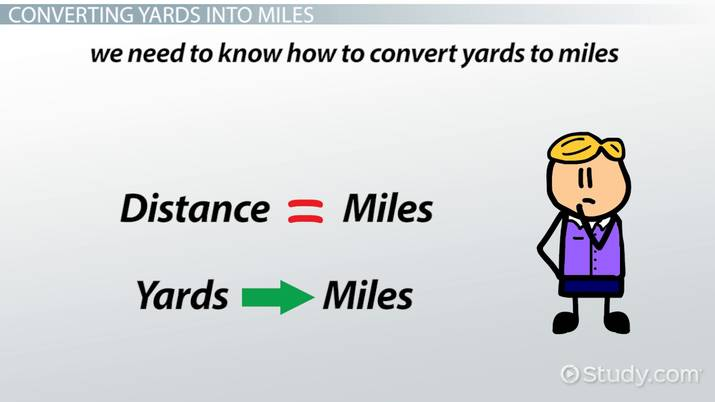Converting Yards to Miles

Lesson Transcript
Instructor: Laura Pennington

Laura received her Master's degree in Pure Mathematics from Michigan State University, and her Bachelor's degree in Mathematics from Grand Valley State University. She has 20 years of experience teaching collegiate mathematics at various institutions.

Measurements in yards can be converted to miles using a simple formula. Practice this conversion through example problems that demonstrate the relationship of a yard to the length of a mile. Updated: 11/12/2021

Converted Yards into Miles

Did you know that a football field in America is 100 yards long, not including the end zones? Have you ever wondered how many football fields you would have to run in order to run a mile, or have you ever wondered how long a football field is in miles?

The Donghai Bridge is located in China, and it is one of the world's longest sea-crossing bridges, measuring in at approximately 35,200 yards long. If you were to travel this bridge by car, how many miles would you have traveled?

There are a number of instances when you may have a distance given to you in yards and you want to know how far that measurement is in miles. To answer these types of questions, we need to know how to convert yards to miles.An error occurred trying to load this video.

Try refreshing the page, or contact customer support.

Coming up next: Semi-Major Axis of an Ellipse

You're on a roll. Keep up the good work!

Replay
Your next lesson will play in 10 seconds
• 0:04 Converting Yards into Miles
• 0:47 Practice Converting…
• 3:13 More Examples of Conversion
• 4:10 Lesson Summary
Save Save

Want to watch this again later?

Timeline
Autoplay
Autoplay
Speed Speed

Practice Converting Yards to Miles

It is common to be more familiar with the distance of one mile as opposed to the distance of one yard. Because of this, it is normal that, if we are given a distance in yards, we want to convert it to miles so we have a better idea of how far it is. For instance, if I told you I ran three miles, you would have a rough idea of how far that is. However, if I were to tell you that I ran 5,280 yards, you may have a harder time picturing how far that is.

The following two statements describe the relationship between yards and miles:

1. There are 1,760 yards in a mile
2. There are 1 / 1,760 = 0.0005681818 miles in a yard

From the second statement we can deduce the following:

• If you have 1 yard, then you have 1 / 1,760 miles
• If you have 2 yards, then you have 2 * (1 / 1,760) = 2 / 1,760 miles
• If you have 3 yards, then you have 3 * (1 / 1,760) = 3 / 1,760 miles

If we were to generalize this pattern, we see that if you have n yards, then you have:We see that to convert yards to miles, we simply need to divide the number of yards by 1,760.

For example, we were wondering how long a football field is in miles. We know that a football field in America is 100 yards long. To figure out how many miles this is, we divide 100 by 1,760. That is:

100 / 1,760 = 0.056818 miles

You would need to run quite a few of these to reach a mile: a little over 17 to be exact.

To unlock this lesson you must be a Study.com Member.

Register to view this lesson

Are you a student or a teacher?